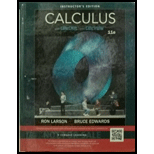Chapter 6.2, Problem 9E### Calculus (MindTap Course List)

11th Edition
Ron Larson + 1 other
ISBN: 9781337275347

#### Solutions

Chapter
Section### Calculus (MindTap Course List)

11th Edition
Ron Larson + 1 other
ISBN: 9781337275347
Textbook Problem

# Solving a Differential Equation In Exercises 3-12, find the general solution of the differential equation. y ' = x y

To determine

To calculate: The differential equation y'=xy and finding a general solution.

Explanation

Formula used: 1xdx=logx

Calculation:

The given equation is y'=xy.

By dividing both sides by y, we get y'y=x

Next, by integrating on both sides, we get y'y=xdydxy=xdyydx=xdyy=

### Still sussing out bartleby?

Check out a sample textbook solution.

See a sample solution

#### The Solution to Your Study Problems

Bartleby provides explanations to thousands of textbook problems written by our experts, many with advanced degrees!

Get Started

## Additional Math Solutions

#### Find more solutions based on key concepts